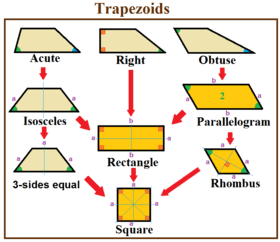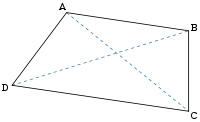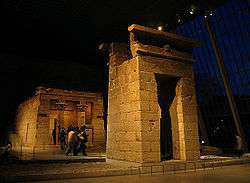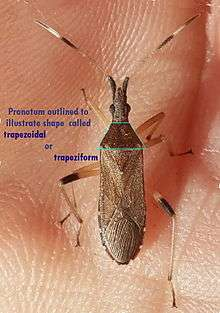# Trapezoid

Trapezoid (AmE)
Trapezium (BrE)Trapezoid
Edges and vertices 4
AreaProperties convex

In Euclidean geometry, a convex quadrilateral with at least one pair of parallel sides is referred to as a trapezoid  (pronounced: /ˈtɹæpəzɔɪd/) in American and Canadian English but as a trapezium in English outside North America. The parallel sides are called the bases of the trapezoid and the other two sides are called the legs or the lateral sides (if they are not parallel; otherwise there are two pairs of bases). A scalene trapezoid is a trapezoid with no sides of equal measure, in contrast to the special cases below.

## Etymology

The term trapezium has been in use in English since 1570, from Late Latin trapezium, from Greek τραπέζιον (trapézion), literally "a little table", a diminutive of τράπεζα (trápeza), "a table", itself from τετράς (tetrás), "four" + πέζα (péza), "a foot, an edge". The first recorded use of the Greek word translated trapezoid (τραπεζοειδή, trapezoeidé, "table-like") was by Marinus Proclus (412 to 485 AD) in his Commentary on the first book of Euclid's Elements.

This article uses the term trapezoid in the sense that is current in the United States and Canada. In many other languages using a word derived from the Greek for this figure, the form closest to trapezium (e.g. French trapèze, Italian trapezio, Spanish trapecio, German Trapez, Russian трапеция) is used.

## Special casesTrapezoid special cases

A right trapezoid (also called right-angled trapezoid) has two adjacent right angles. Right trapezoids are used in the trapezoidal rule for estimating areas under a curve.

An acute trapezoid has two adjacent acute angles on its longer base edge, while an obtuse trapezoid has one acute and one obtuse angle on each base.

An acute trapezoid is also an isosceles trapezoid, if its sides (legs) have the same length, and the base angles have the same measure. It has reflection symmetry.

An obtuse trapezoid with two pairs of parallel sides is a parallelogram. A parallelogram has central 2-fold rotational symmetry (or point reflection symmetry).

A Saccheri quadrilateral is similar to a trapezoid in the hyperbolic plane, with two adjacent right angles, while it is a rectangle in the Euclidean plane. A Lambert quadrilateral in the hyperbolic plane has 3 right angles.

A tangential trapezoid is a trapezoid that has an incircle.

## Inclusive vs exclusive definition

There is some disagreement whether parallelograms, which have two pairs of parallel sides, should be regarded as trapezoids. Some define a trapezoid as a quadrilateral having only one pair of parallel sides (the exclusive definition), thereby excluding parallelograms. Others define a trapezoid as a quadrilateral with at least one pair of parallel sides (the inclusive definition), making the parallelogram a special type of trapezoid. The latter definition is consistent with its uses in higher mathematics such as calculus. The former definition would make such concepts as the trapezoidal approximation to a definite integral ill-defined. This article uses the inclusive definition and considers parallelograms as special cases of a trapezoid. This is also advocated in the taxonomy of quadrilaterals.

Under the inclusive definition, all parallelograms (including rhombuses, rectangles and squares) are trapezoids. Rectangles have mirror symmetry on mid-edges; rhombuses have mirror symmetry on vertices, while squares have mirror symmetry on both mid-edges and vertices.

## Condition of existence

Four lengths a, c, b, d can constitute the consecutive sides of a non-parallelogram trapezoid with a and b parallel only whenThe quadrilateral is a parallelogram when, but it is an ex-tangential quadrilateral (which is not a trapezoid) when.:p. 35

## Characterizations

Given a convex quadrilateral, the following properties are equivalent, and each implies that the quadrilateral is a trapezoid:

• It has two adjacent angles that are supplementary, that is, they add up to 180 degrees.
• The angle between a side and a diagonal is equal to the angle between the opposite side and the same diagonal.
• The diagonals cut each other in mutually the same ratio (this ratio is the same as that between the lengths of the parallel sides).
• The diagonals cut the quadrilateral into four triangles of which one opposite pair are similar.
• The diagonals cut the quadrilateral into four triangles of which one opposite pair have equal areas.:Prop.5
• The product of the areas of the two triangles formed by one diagonal equals the product of the areas of the two triangles formed by the other diagonal.:Thm.6
• The areas S and T of some two opposite triangles of the four triangles formed by the diagonals satisfy the equationwhere K is the area of the quadrilateral.:Thm.8
• The midpoints of two opposite sides and the intersection of the diagonals are collinear.:Thm.15
•:p. 25
• The cosines of two adjacent angles sum to 0, as do the cosines of the other two angles.:p. 25
• The cotangents of two adjacent angles sum to 0, as do the cotangents of the other two adjacent angles.:p. 26
• One bimedian divides the quadrilateral into two quadrilaterals of equal areas.:p. 26
• Twice the length of the bimedian connecting the midpoints of two opposite sides equals the sum of the lengths of the other sides.:p. 31

Additionally, the following properties are equivalent, and each implies that opposite sides a and b are parallel:

• The consecutive sides a, c, b, d and the diagonals p, q satisfy the equation:Cor.11• The distance v between the midpoints of the diagonals satisfies the equation:Thm.12## Midsegment and height

The midsegment (also called the median or midline) of a trapezoid is the segment that joins the midpoints of the legs. It is parallel to the bases. Its length m is equal to the average of the lengths of the bases a and b of the trapezoid,The midsegment of a trapezoid is one of the two bimedians (the other bimedian divides the trapezoid into equal areas).

The height (or altitude) is the perpendicular distance between the bases. In the case that the two bases have different lengths (ab), the height of a trapezoid h can be determined by the length of its four sides using the formulawhere c and d are the lengths of the legs.

## Area

The area K of a trapezoid is given bywhere a and b are the lengths of the parallel sides, h is the height (the perpendicular distance between these sides), and m is the arithmetic mean of the lengths of the two parallel sides. In 499 AD Aryabhata, a great mathematician-astronomer from the classical age of Indian mathematics and Indian astronomy, used this method in the Aryabhatiya (section 2.8). This yields as a special case the well-known formula for the area of a triangle, by considering a triangle as a degenerate trapezoid in which one of the parallel sides has shrunk to a point.

The 7th-century Indian mathematician Bhāskara I derived the following formula for the area of a trapezoid with consecutive sides a, c, b, d:where a and b are parallel and b > a. This formula can be factored into a more symmetric versionWhen one of the parallel sides has shrunk to a point (say a = 0), this formula reduces to Heron's formula for the area of a triangle.

Another equivalent formula for the area, which more closely resembles Heron's formula, iswhereis the semiperimeter of the trapezoid. (This formula is similar to Brahmagupta's formula, but it differs from it, in that a trapezoid might not be cyclic (inscribed in a circle). The formula is also a special case of Bretschneider's formula for a general quadrilateral).

From Bretschneider's formula, it follows thatThe line that joins the midpoints of the parallel sides, bisects the area.

## DiagonalsThe lengths of the diagonals arewhere a and b are the bases, c and d are the other two sides, and a < b.

If the trapezoid is divided into four triangles by its diagonals AC and BD (as shown on the right), intersecting at O, then the area ofAOD is equal to that ofBOC, and the product of the areas ofAOD andBOC is equal to that ofAOB andCOD. The ratio of the areas of each pair of adjacent triangles is the same as that between the lengths of the parallel sides.

Let the trapezoid have vertices A, B, C, and D in sequence and have parallel sides AB and DC. Let E be the intersection of the diagonals, and let F be on side DA and G be on side BC such that FEG is parallel to AB and CD. Then FG is the harmonic mean of AB and DC:The line that goes through both the intersection point of the extended nonparallel sides and the intersection point of the diagonals, bisects each base.

## Other properties

The center of area (center of mass for a uniform lamina) lies along the line segment joining the midpoints of the parallel sides, at a perpendicular distance x from the longer side b given byThe center of area divides this segment in the ratio (when taken from the short to the long side):p. 862If the angle bisectors to angles A and B intersect at P, and the angle bisectors to angles C and D intersect at Q, then## More on terminology

The term trapezoid was once defined as a quadrilateral without any parallel sides in Britain and elsewhere. (The Oxford English Dictionary says "Often called by English writers in the 19th century".) According to the Oxford English Dictionary, the sense of a figure with no sides parallel is the meaning for which Proclus introduced the term "trapezoid". This is retained in the French trapézoïde (), German Trapezoid, and in other languages. However, this particular sense is considered obsolete.

A trapezium in Proclus' sense is a quadrilateral having one pair of its opposite sides parallel. This was the specific sense in England in 17th and 18th centuries, and again the prevalent one in recent use outside North America. A trapezium as any quadrilateral more general than a parallelogram is the sense of the term in Euclid.

Confusingly, the word trapezium was sometimes used in England from c. 1800 to c. 1875, to denote an irregular quadrilateral having no sides parallel. This is now obsolete in England, but continues in North America. However this shape is more usually (and less confusingly) just called an irregular quadrilateral.

## Application in geometry

The crossed ladders problem is the problem of finding the distance between the parallel sides of a right trapezoid, given the diagonal lengths and the distance from the perpendicular leg to the diagonal intersection.

## ArchitectureIn architecture the word is used to refer to symmetrical doors, windows, and buildings built wider at the base, tapering toward the top, in Egyptian style. If these have straight sides and sharp angular corners, their shapes are usually isosceles trapezoids. This was the standard style for the doors and windows of the Inca.

## Application in biologyExample of a trapeziform pronotum outlined on a spurge bug

In morphology, taxonomy and other descriptive disciplines in which a term for such shapes is necessary, terms such as trapezoidal or trapeziform commonly are useful in descriptions of particular organs or forms.

2. Oxford English Dictionary entry at trapezoid.
3. "American School definition from "math.com"". Retrieved 2008-04-14.
4. Trapezoids, . Retrieved 2012-02-24.
5. Ask Dr. Math (2008), "Area of Trapezoid Given Only the Side Lengths".
6. Martin Josefsson, "Characterizations of trapezoids", Forum Geometricorum, 13 (2013) 23-35.
7. T. K. Puttaswamy, Mathematical achievements of pre-modern Indian mathematicians, Elsevier, 2012, p. 156.
8. GoGeometry, . Retrieved 2012-07-08.
9. Owen Byer, Felix Lazebnik and Deirdre Smeltzer, Methods for Euclidean Geometry, Mathematical Association of America, 2010, p. 55.
10. efunda, General Trapezoid, . Retrieved 2012-07-09.
11. Tom M. Apostol and Mamikon A. Mnatsakanian (December 2004). "Figures Circumscribing Circles" (PDF). American Mathematical Monthly: 853–863. Retrieved 2016-04-06.
12. Oxford English Dictionary entries for trapezoid and trapezium.
13. Chambers 21st Century Dictionary Trapezoid
14. "1913 American definition of trapezium". Merriam-Webster Online Dictionary. Retrieved 2007-12-10.
15. http://gogeometry.com/MachuPicchu.htm
16. John L. Capinera (11 August 2008). Encyclopedia of Entomology. Springer Science & Business Media. pp. 386, 1062, 1247. ISBN 978-1-4020-6242-1.